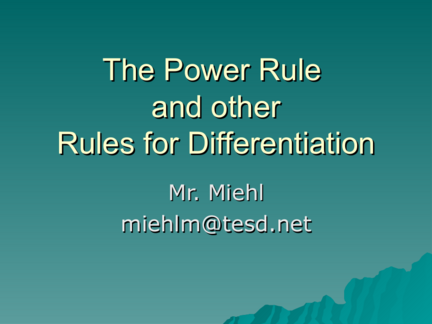# The Power Rule and other Rules for DifferentiationContributed by:OBJECTIVE:
To differentiate functions using the power rule, constant rule, constant multiple rules, and sum and difference rules.
1. The Power Rule
and other
Rules for Differentiation
Mr. Miehl
[email protected]
2. Rules for Differentiation
Taking the derivative by using the definition is a lot of work.
Perhaps there is an easy way to find the derivative.
3. Objective
 Todifferentiate functions using the
power rule, constant rule, constant
multiple rule, and sum and difference
rules.
4. The Derivative is …
 Used to find the “slope” of a function at a point.
 Used to find the “slope of the tangent line” to
the graph of a function at a point.
 Used to find the “instantaneous rate of change”
of a function at a point.
 Computed by finding the limit of the difference
quotient as ∆x approaches 0. (Limit Definition)
5. Notations for the
Derivative of a Function
f '( x) “f prime of x”
y' “y prime”
“the derivative of y with respect to x”
is a noun.
is a verb. “Take the derivative with respect to x…”
6. Rules for Differentiation
 Differentiation
is the process of
computing the derivative of a
function.
 Differentiate.
 Derive.
 Find the derivative of…
7. Video Clip from
The Power Rule
8. Rules for Differentiation
 Working with the definition of the
derivative is important because it
helps you really understand what the
derivative means.
9. The Power Rule
d N
[ x ] Nx N  1 , N is any real number
d
[ x] 1
dx
10. The Constant Rule
d
[c] 0, c is a constant
dx
 The derivative of a constant function
is zero.
11. The Constant Multiple Rule
d
[c  f ( x)  ] c  f '( x)  , c is a constant
dx
 Thederivative of a constant times a
function is equal to the constant
times the derivative of the function.
12. The Sum and Difference Rules
d
[ f ( x)  g ( x)]  f '( x)  g '( x)
dx
The derivative of a sum is the sum of the derivatives.
d
[ f ( x)  g ( x)]  f '( x)  g '( x)
dx
The derivative of a difference is the difference of the derivatives.
13. Constant Rule
 Find the derivative of:
f ( x) 7
f '( x) 0
y  3
dy
0 or y ' 0
dx
14. Power Rule
 Differentiate:
f ( x) x 3 g ( x) x100
2 99
f '( x) 3 x g '( x) 100 x
y x 9
dy 8
9 x
dx
15. Constant Multiple Rule
 Find the derivative of:
1
y 2 x 3
dy 2
2  1
3 x
 3

dx
dy 2
 2
dx 3 x 3
16. Constant Multiple Rule
 Find the derivative of:
4x2 4 2
f ( x)   x
5 5
f '( x)  54  2x 
8
f '( x)  x
5
17. Constant Multiple Rule
 Find the derivative of:
7
g ( x) 5 x
6
g '( x) 35 x
18. Rewriting Before Differentiating
Function Rewrite Differentiate Simplify
5 5 5 15
f ( x)  3 f ( x)  x 3 f '( x )  (  3 x  4 ) f '( x ) 
2x 2 2 2 x4
19. Rewriting Before Differentiating
Function Rewrite Differentiate Simplify
7 7 2 7 14
g( x )   2 g( x )  x g '( x )  (2 x ) g '( x )  x
3x 3 3 3
20. Rewriting Before Differentiating
Function Rewrite Differentiate Simplify
1 1  12 1
h( x )  x h( x )  x 2 h '( x )  x h '( x )  1
2 2x 2
21. Rewriting Before Differentiating
Function Rewrite Differentiate Simplify
1
j( x )  2
1 2x
3
1  2  53  1
j( x )  j '( x )    x  j '( x )  5
3
2 x 2
2 3  3x 3
1  23
j( x )  x
2
22. Sum & Difference Rules
 Differentiate:
f ( x) 5 x 2  7 x  6
f '( x) 10x 7
g ( x) 4 x 6  3 x 5  10 x 2  5 x  16
g '( x) 24x 5  15x 4  20x 5
23. Conclusion
 Notations for the derivative:
f '( x) dy
y'
dx
 The derivative of a constant is zero.
 To find the derivative of f (x) = xN
1. Pull a copy of the exponent out in
front of the term.
2. Subtract one from the exponent.